C# 8 introduces some new pattern types. In this blog I want to look at the new positional patterns. Some parts of the documentation use a different name, calling them deconstruction patterns, and they do look very similar to the deconstructing assignments we were exploring earlier in this series.

Here's a positional pattern (aka deconstruction pattern) in context:

``````if (p is Point (int x, _))
{
return x;
}
``````

For clarity, here's the pattern part:

``````Point (int x, _)
``````

And this is how the compiler presents this through the Roslyn API: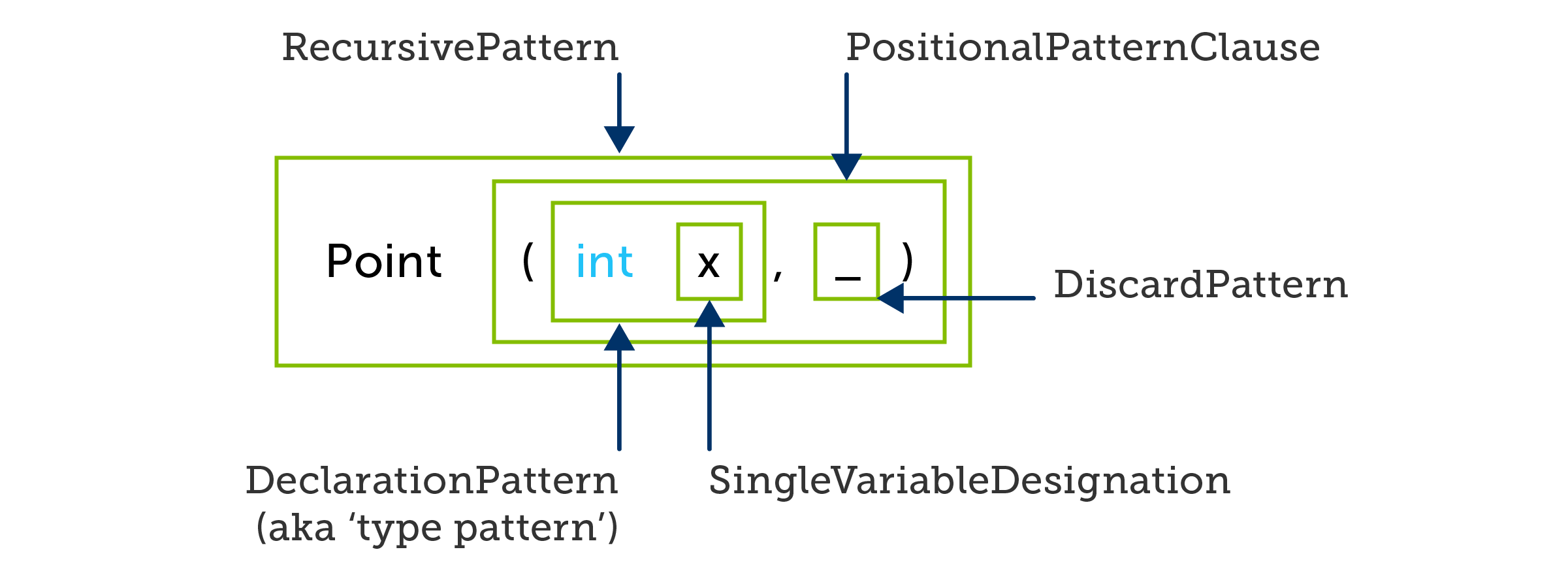When this pattern matches, it introduces a new variable x which takes on the first value from deconstruction. There's an obvious resemblance to the assignment example from the preceding blog in this series:

``````(int x, _) = p;
``````

Or, as Roslyn sees it: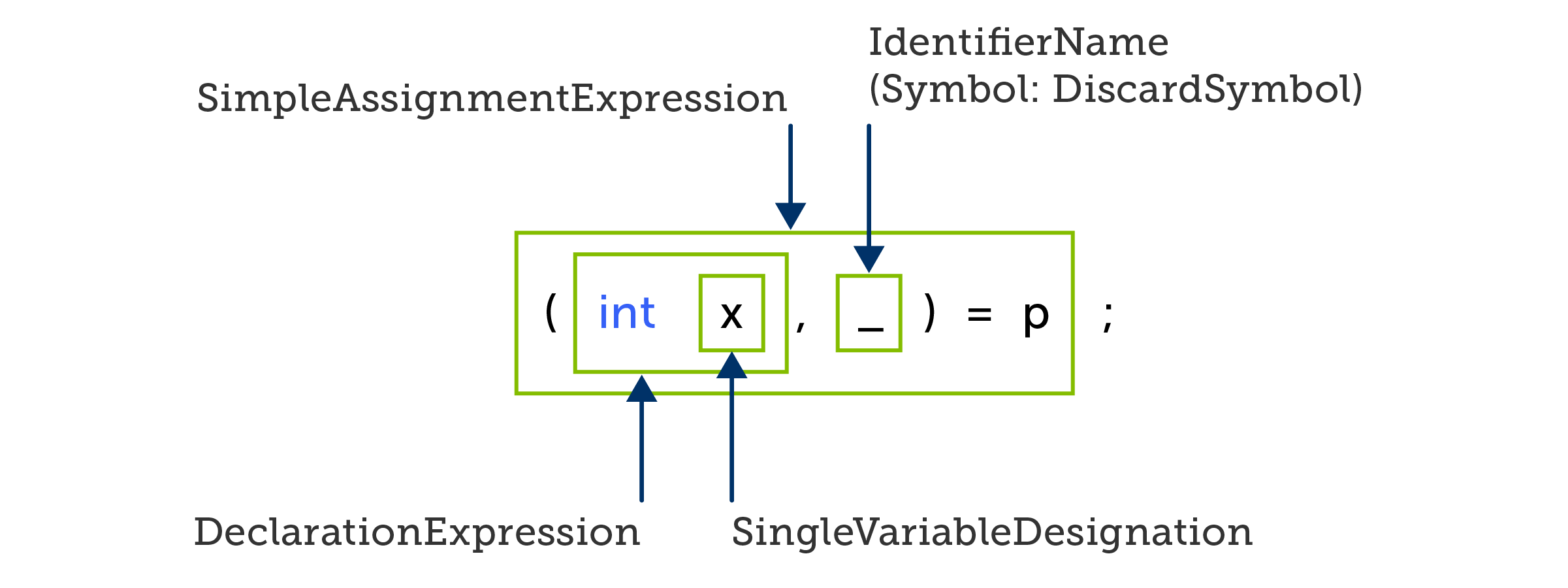The main difference is that patterns can fail to match at runtime. If at runtime `p` did not refer to a `Point`, the pattern would evaluate to `false`, and body of the `if` statement above would not run, but as long as `p`'s static type means that it could conceivably refer to a `Point`, the code will at least compile. By contrast, deconstructing assignments always run, so the compiler would only allow the preceding example if the static type of `p` offers a suitable deconstructor.

For example, if `p` here is a variable of type `object`, the pattern example will compile, but the deconstructing assignment will not.

The visual similarity of the part in parentheses makes it tempting to think of a positional pattern as being essentially the same as a deconstructing assignment, but with a runtime type check instead of a compile time one. However, this would be to fall into the trap set by these faux amis.

We saw earlier in this series that a deconstructing assignment can either declare a new variable in each non-discard position, or it can refer to existing variables. The same is true for positional/deconstructing patterns. However, it means something quite different. Take this example:

``````const int x = 20;
int _ = 10;
if (p is Point (x, _))
{
return _;
}
``````

The first clue that something is amiss is the `const` qualifier on the declaration of `x`. If you try to use a deconstructing assignment with this declaration of `x`, the compiler will reject it because you cannot assign into a `const` variable outside of its declaration. By contrast, this pattern example requires that `const` if it is to compile. This tells us that despite the superficial similarities between deconstructing assignments and positional patterns, they're really not the same.

It turns out that when you put an existing variable into some position in a positional pattern, the compiler interprets this as a nested constant pattern. Here's how Roslyn sees it: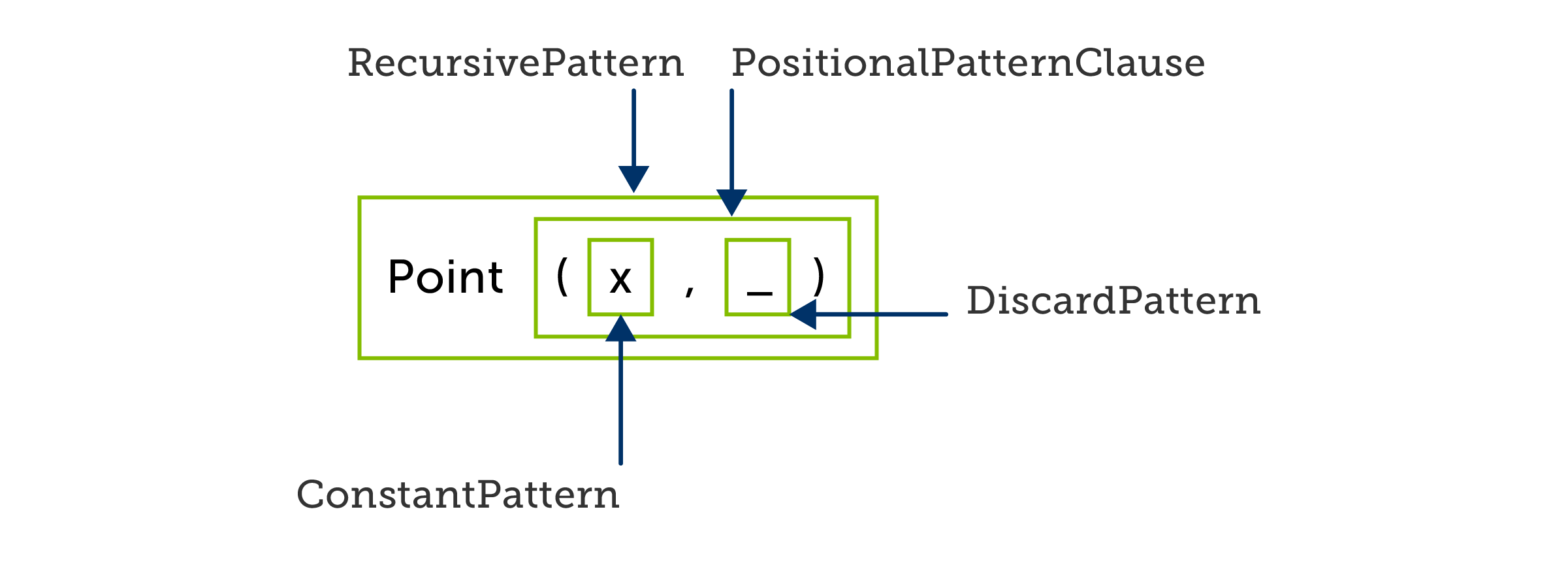That whole pattern will only match for instances of `Point` where the first value from the deconstructor has a certain constant value (20 in this case).

With assignments, changing from the form that declares the variable inline:

``````(int x, _) = p;
``````

to the form where you use a predeclared variable:

``````int x;
(x, _) = p;
``````

doesn't change the basic behaviour. They look slightly different through the Roslyn API. Here's the first one: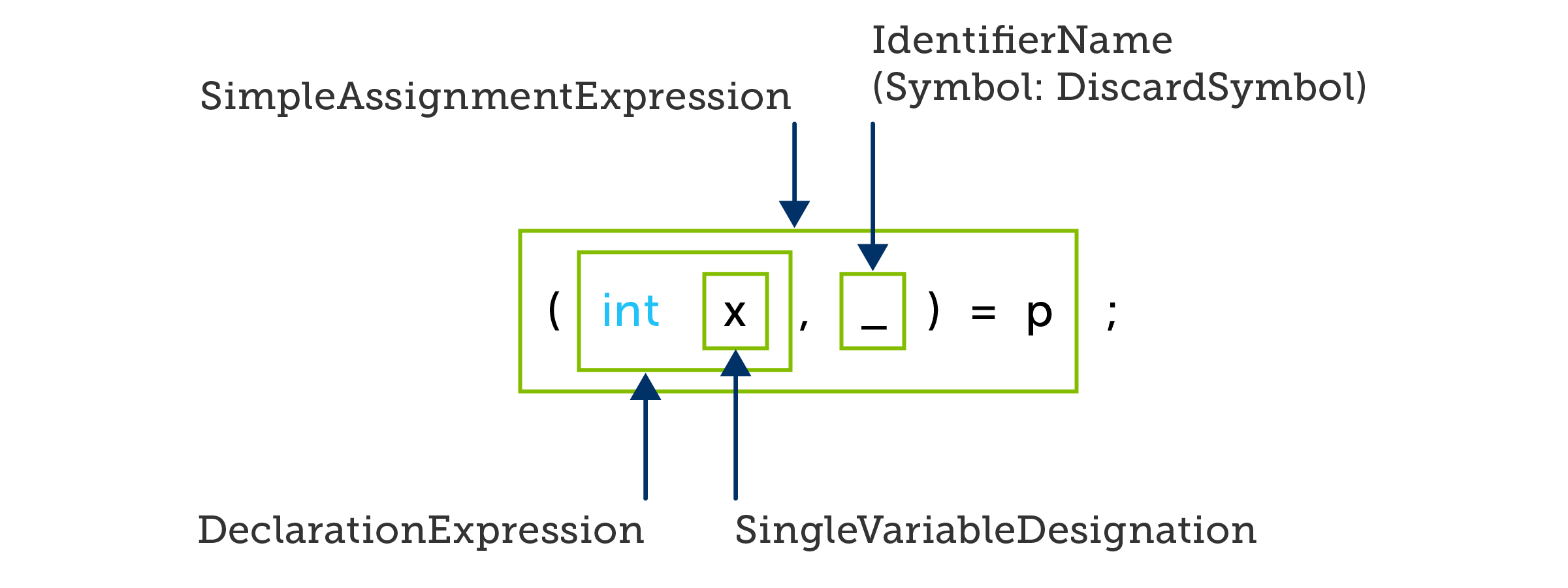and here's the second: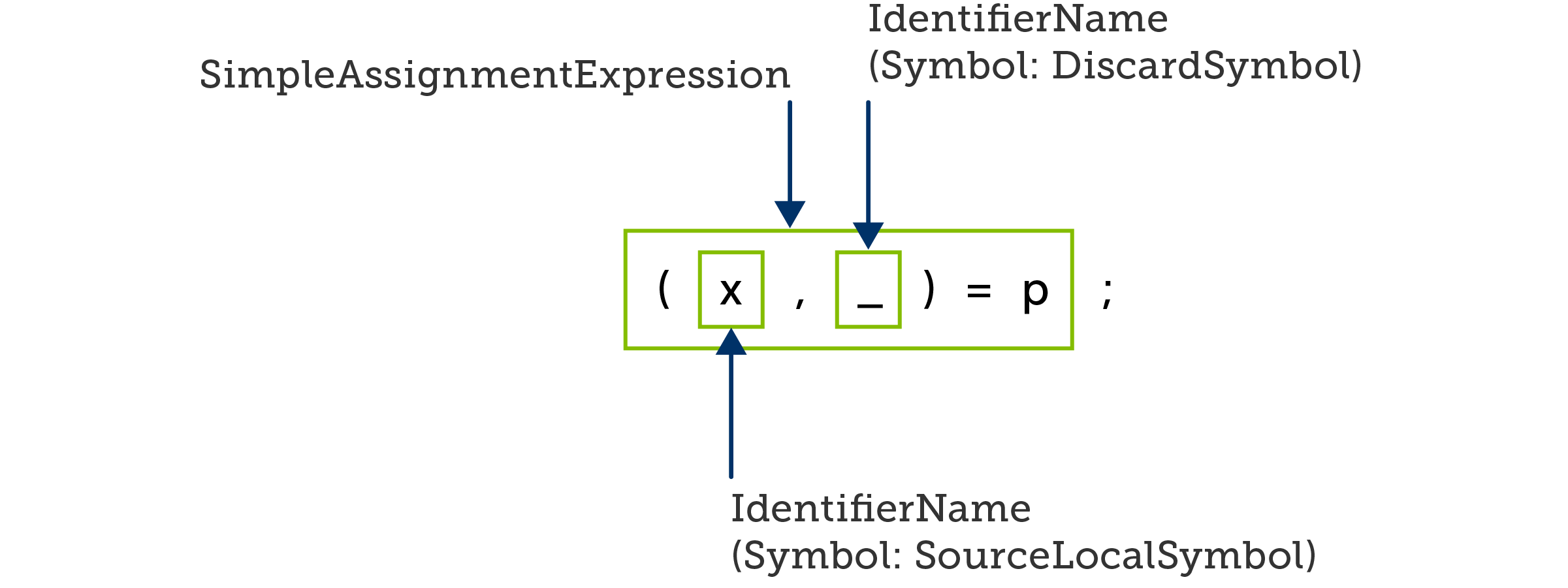In either case we're extracting a value and putting it in x. But in the pattern example, it fundamentally changes the behaviour. In the original form:

``````Point (int x, _)
``````

we are deconstructing a `Point` and storing one of its values in x, whereas if we use a predeclared variable:

``````Point (x, _)
``````

we are defining a pattern that only matches `Point`s where the first deconstructed value is the same as the constant `x`, and which does not extract any of the deconstructed values from that `Point` into variables.

The whole example in which I showed that pattern has some further weirdness. Here it is again:

``````const int x = 20;
int _ = 10;
if (p is Point (x, _))
{
return _;
}
``````

Notice that I defined a local variable named _. Interestingly although both x and _ appear in the pattern, the compiler only insists that x be `const`. That's because despite the superficial similarity between deconstructing assignments and positional patterns, they are faux amis. Whereas a deconstructing assignment can treat an _ as being either an assignment into an existing variable named _, or a discard, depending on whether there is a variable named _ in scope, it turns out that when you write _ as a nested pattern inside a positional pattern, C# will never interpret it as a variable, even when a variable named _ is in scope.

This means that the `return` statement in this example has the potential to surprise. If you were familiar with C# 7.0's deconstructing assignments and this was the first time you'd seen a positional pattern, you might have expected this to extract the second deconstructed value from `Point` into the local variable _, and then to return it. But that isn't what happens. The local variable _ will continue to have its value of 10 no matter what's in the `Point`, because the pattern doesn't use the _ variable, despite how it might look.

When _ appears as a nested pattern of any recursive pattern, it is always treated as a discard pattern, one of the new pattern types introduced in C# 8. Syntactically and semantically, discard patterns are different from either `out` discards or discards in variable designations such as deconstructing assignments. And just to add to the confusion, it is possible for a discard designation (not in itself a pattern) to appear inside a pattern:

``````Point (x, int _))
``````

That is a positional pattern with two nested patterns. The first nested pattern (x) is a constant pattern, and the second (`int _`) is a declaration pattern with a discard designation (_). Here's the Roslyn view: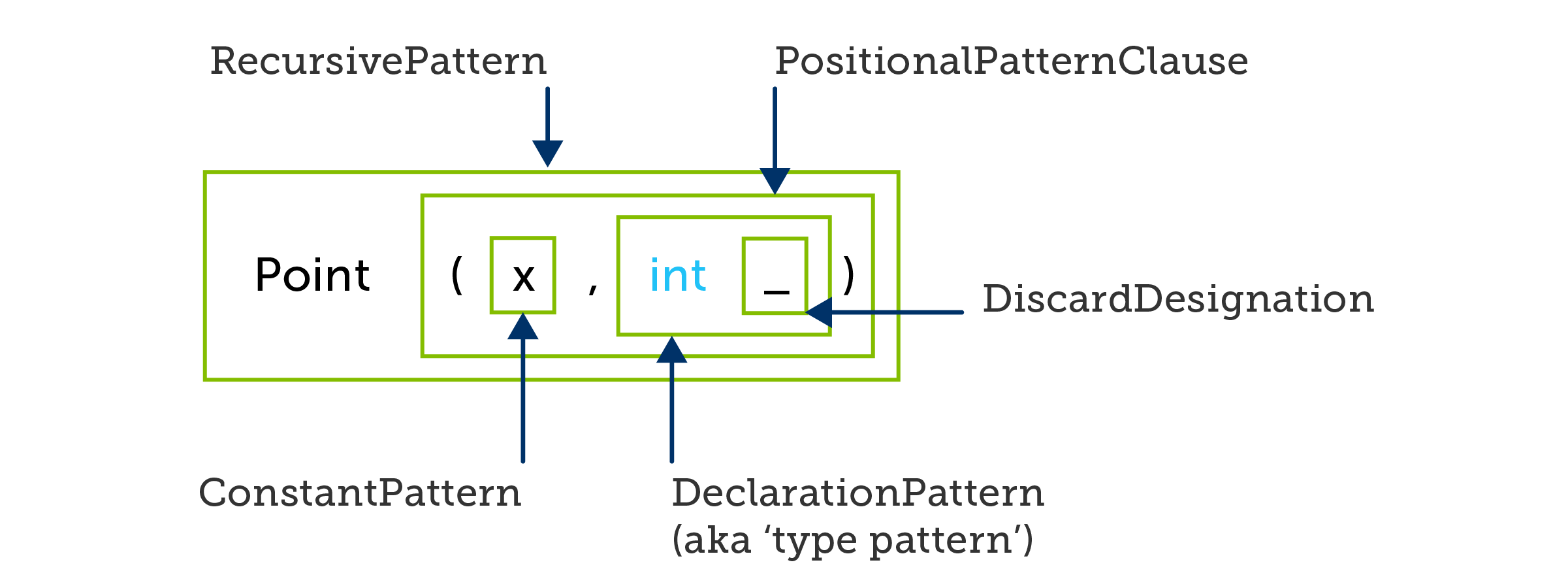So although there's a discard here, there's no discard pattern. The discard pattern is only in use if _ is the whole of the pattern. And if you're wondering why the _ doesn't count as a nested pattern of `int _` just as the `int _` is a nested pattern inside the whole positional pattern, it's because declaration patterns are not recursive: they cannot contain nested patterns. (That's why it's a `DiscardDesignation` here, where earlier we saw a `DiscardPattern`.)

This is the basic problem with faux amis: they make it tricky to work out exactly what you're looking at, because there are numerous language constructs with various superficial similarities, but quite different meanings.

Next time, I will get to the example that I originally stumbled across.

### Ian Griffiths

#### Technical Fellow IIan has worked in various aspects of computing, including computer networking, embedded real-time systems, broadcast television systems, medical imaging, and all forms of cloud computing. Ian is a Technical Fellow at endjin, and Microsoft MVP in Developer Technologies. He is the author of O'Reilly's Programming C# 10.0, and has written Pluralsight courses on WPF (and here) and the TPL. He's a maintainer of Reactive Extensions for .NET, Reaqtor, and endjin's 50+ open source projects. Technology brings him joy.